# NCERT Exemplar Class 11 Physics Solutions for Chapter 15 - Waves

NCERT Exemplar Solutions for Class 11 Physics Chapter 15 Waves are provided here in PDF for easy access and download for the students. This solution provides answers to the questions in the NCERT Exemplar book that helps in understanding the topic from the exam point of view.

NCERT Exemplar Class 11 Physics Chapter 15 Waves provides important questions, problems and solutions, MCQs, short and long answer questions, worksheets and exercises, which will help you to prepare NCERT notes on Waves and, in due course, save you a lot of time when you revise the topics during Class 11 annual exam.

A wave can be defined as a disturbance which transfers energy through time and space. They are of two types, mechanical and electromagnetic waves. Sound waves usually propagate through the air molecules by colliding with the neighbours.

## Download the PDF of NCERT Exemplar Class 11 Physics Chapter 15 Waves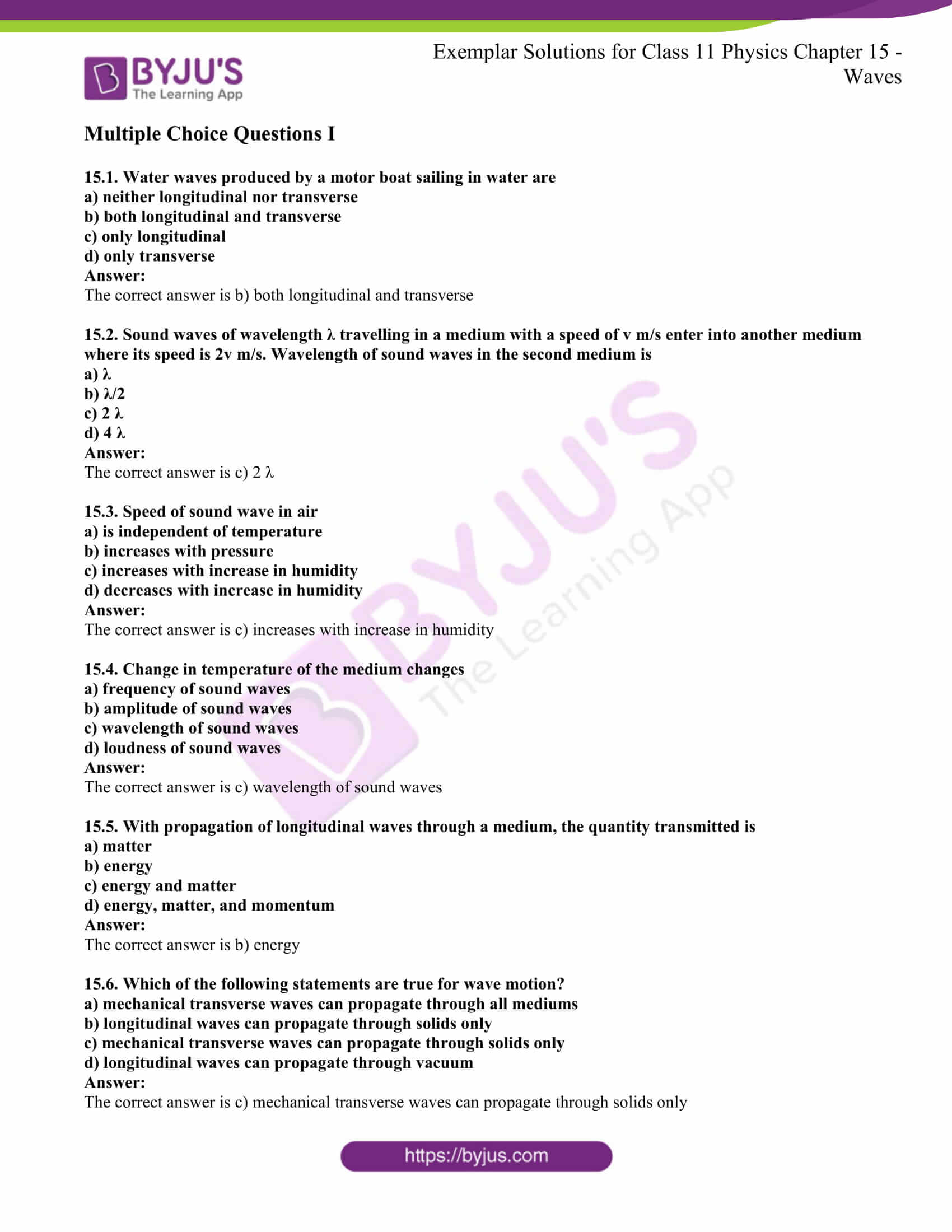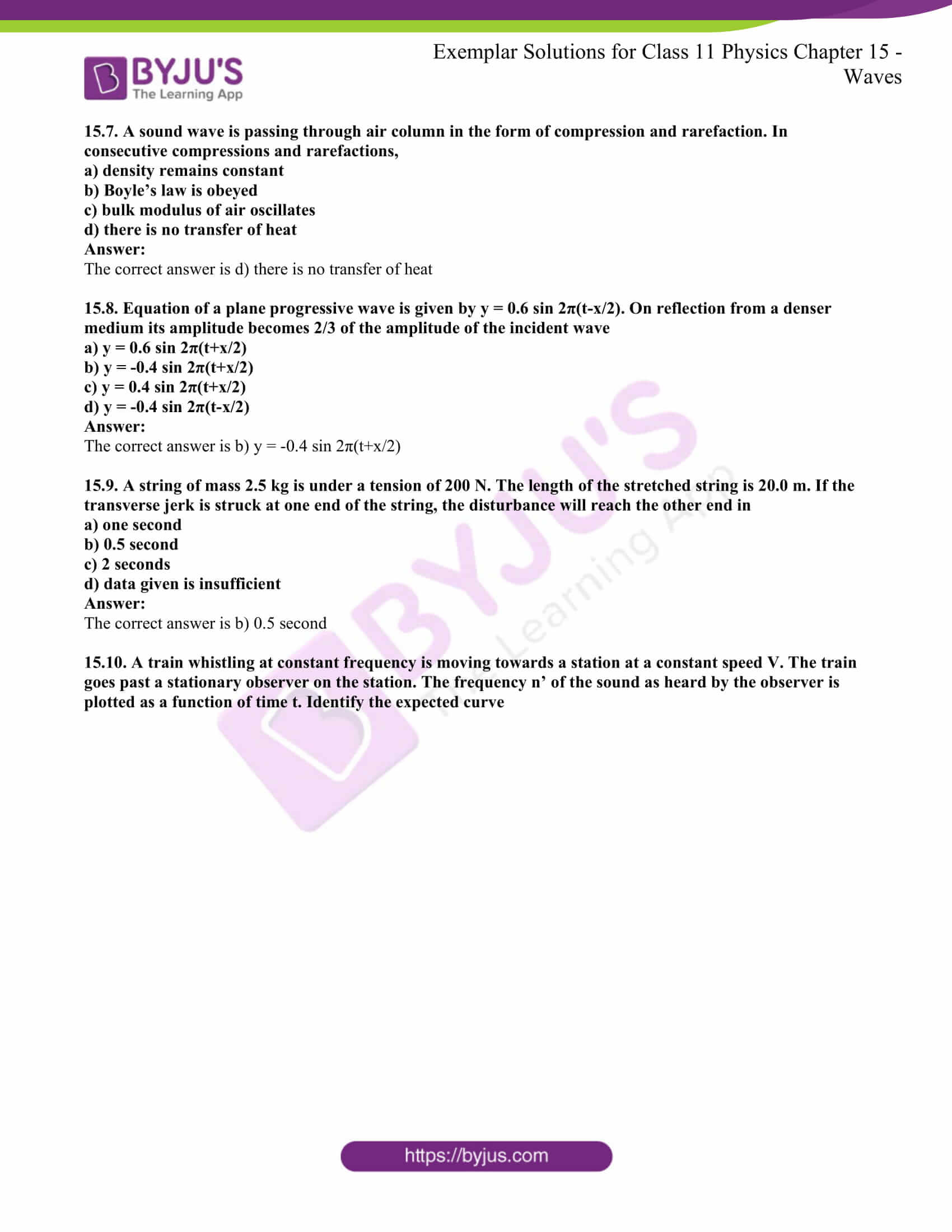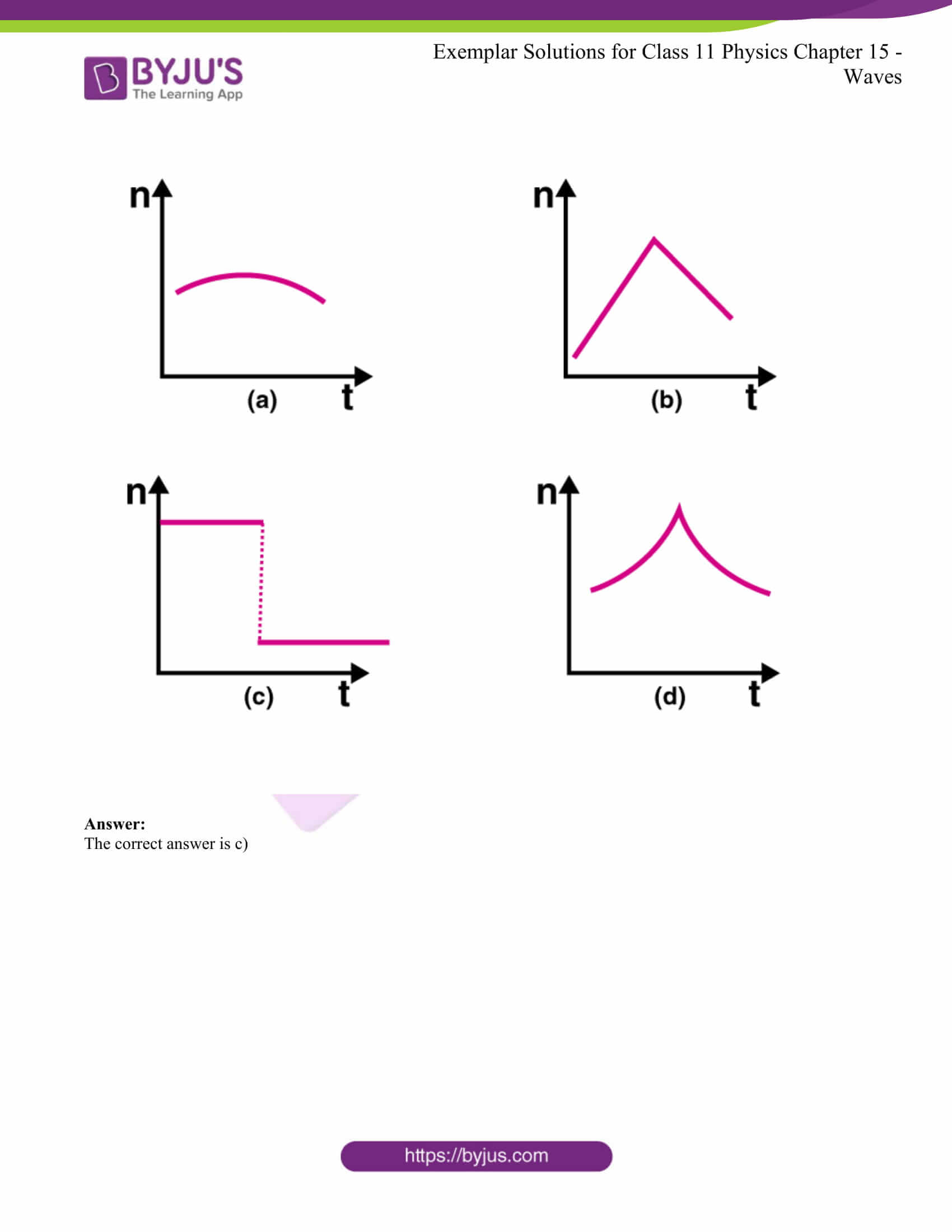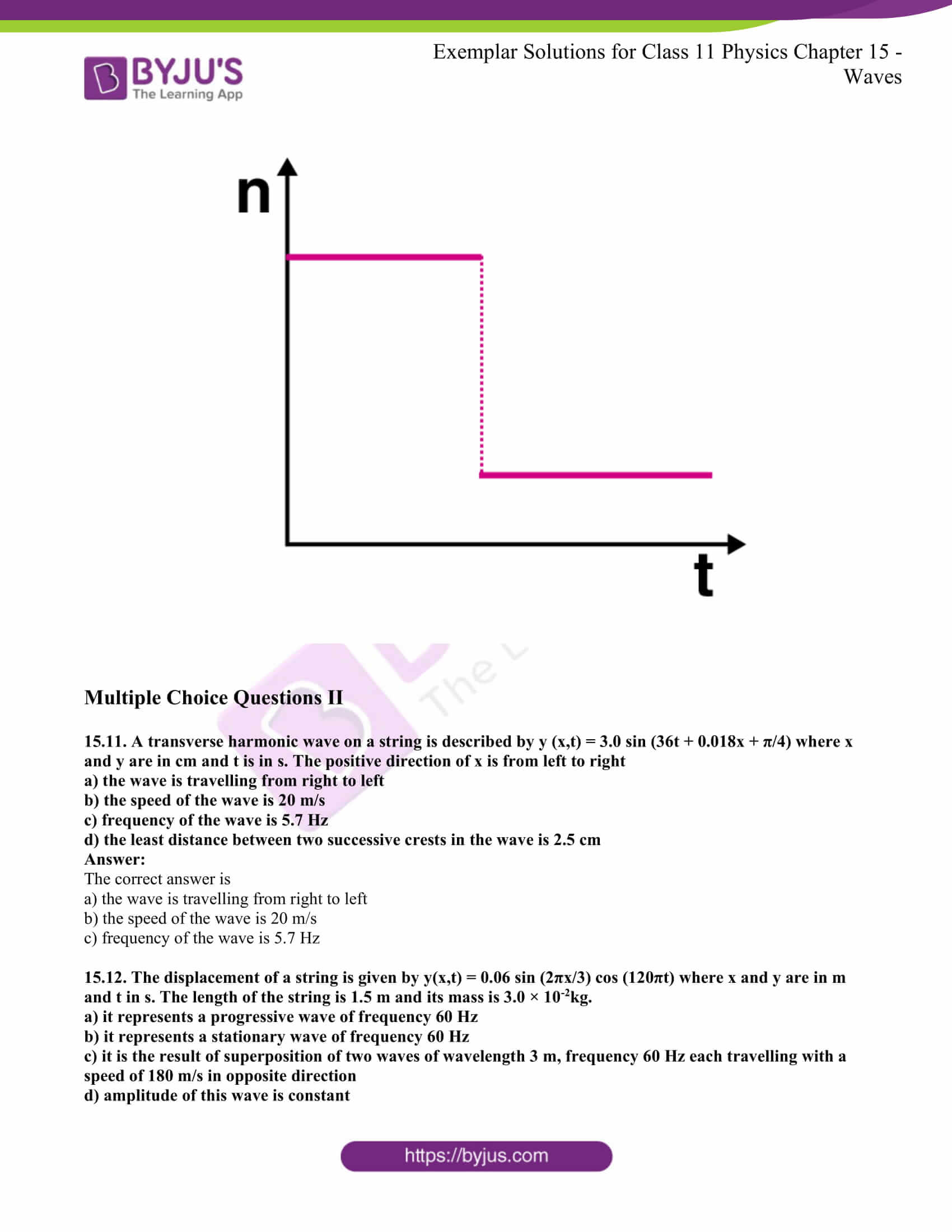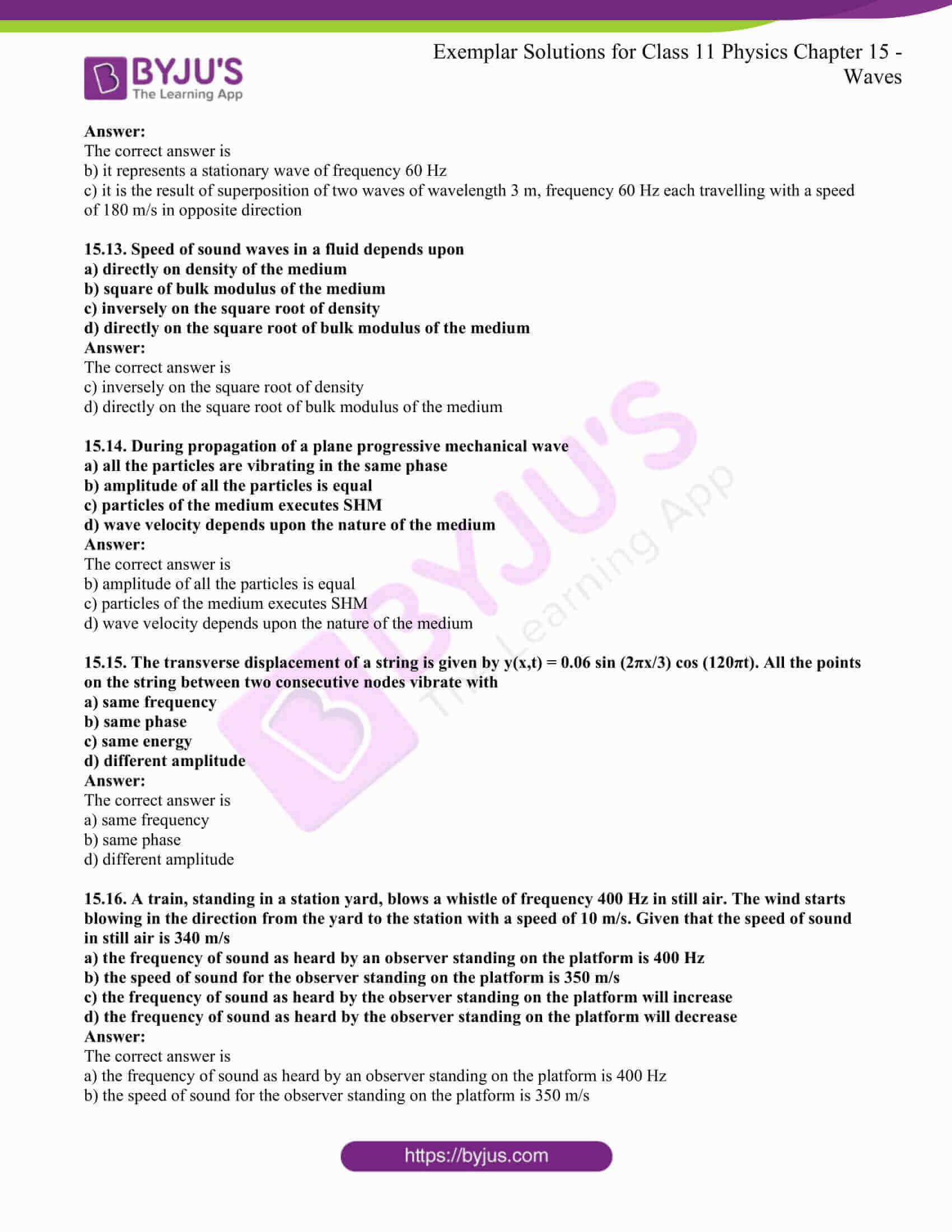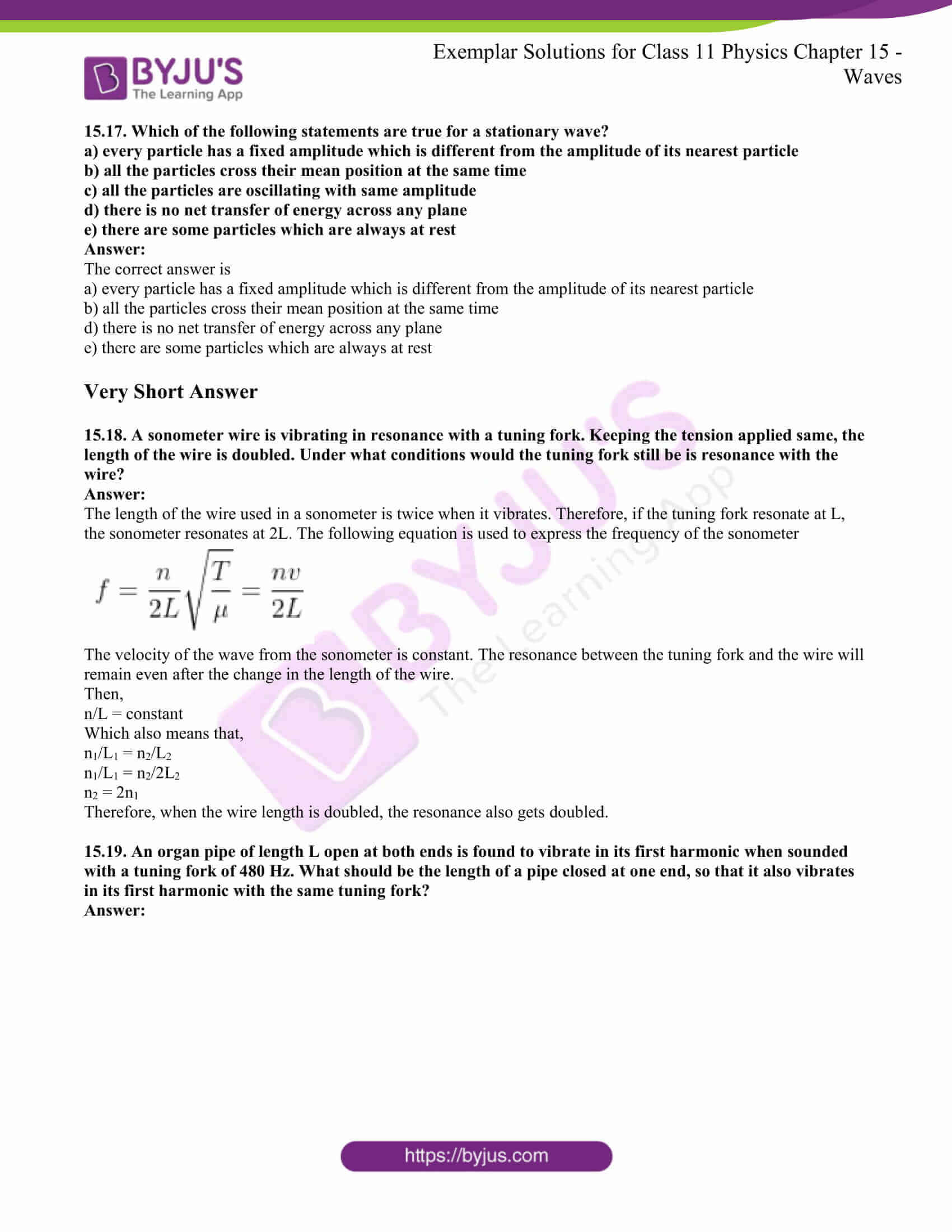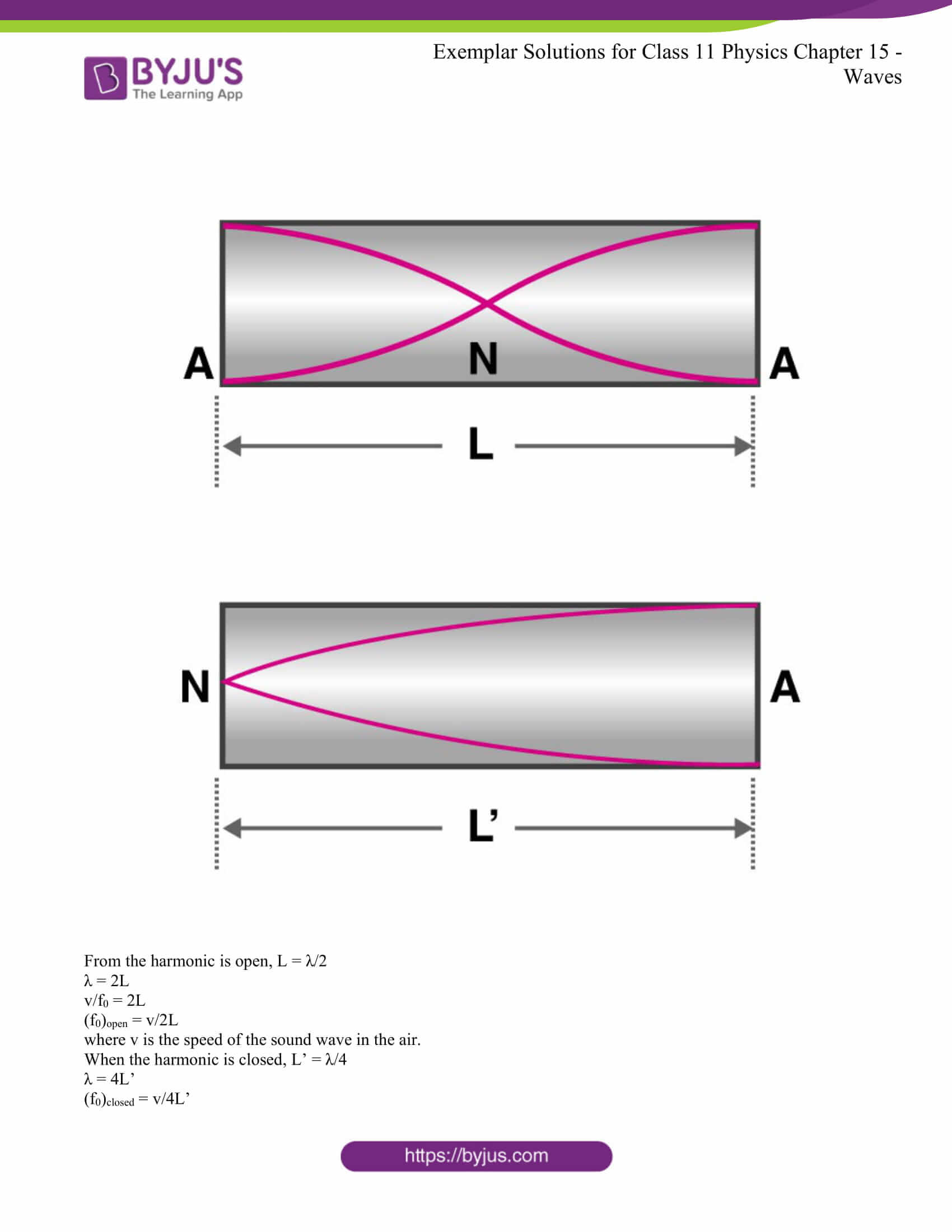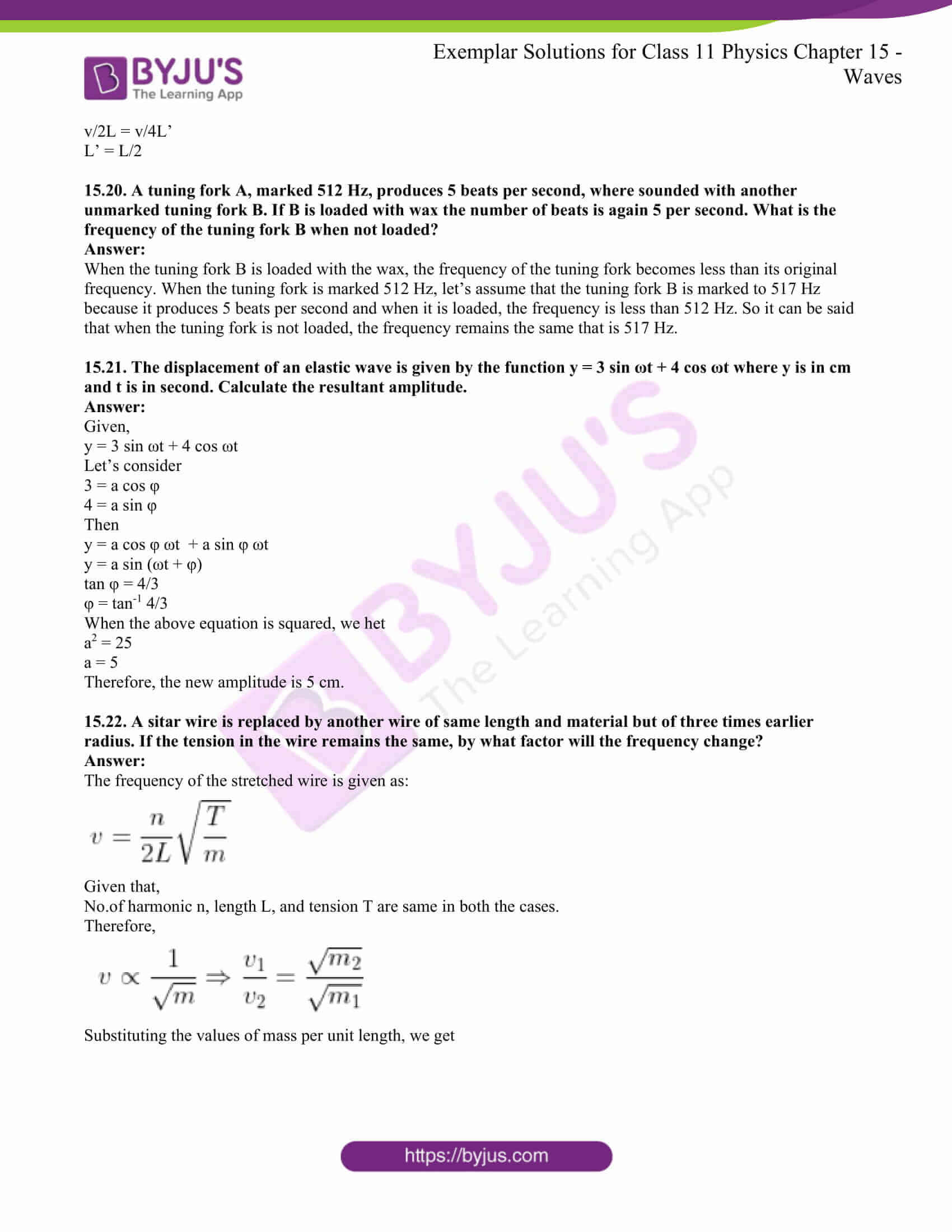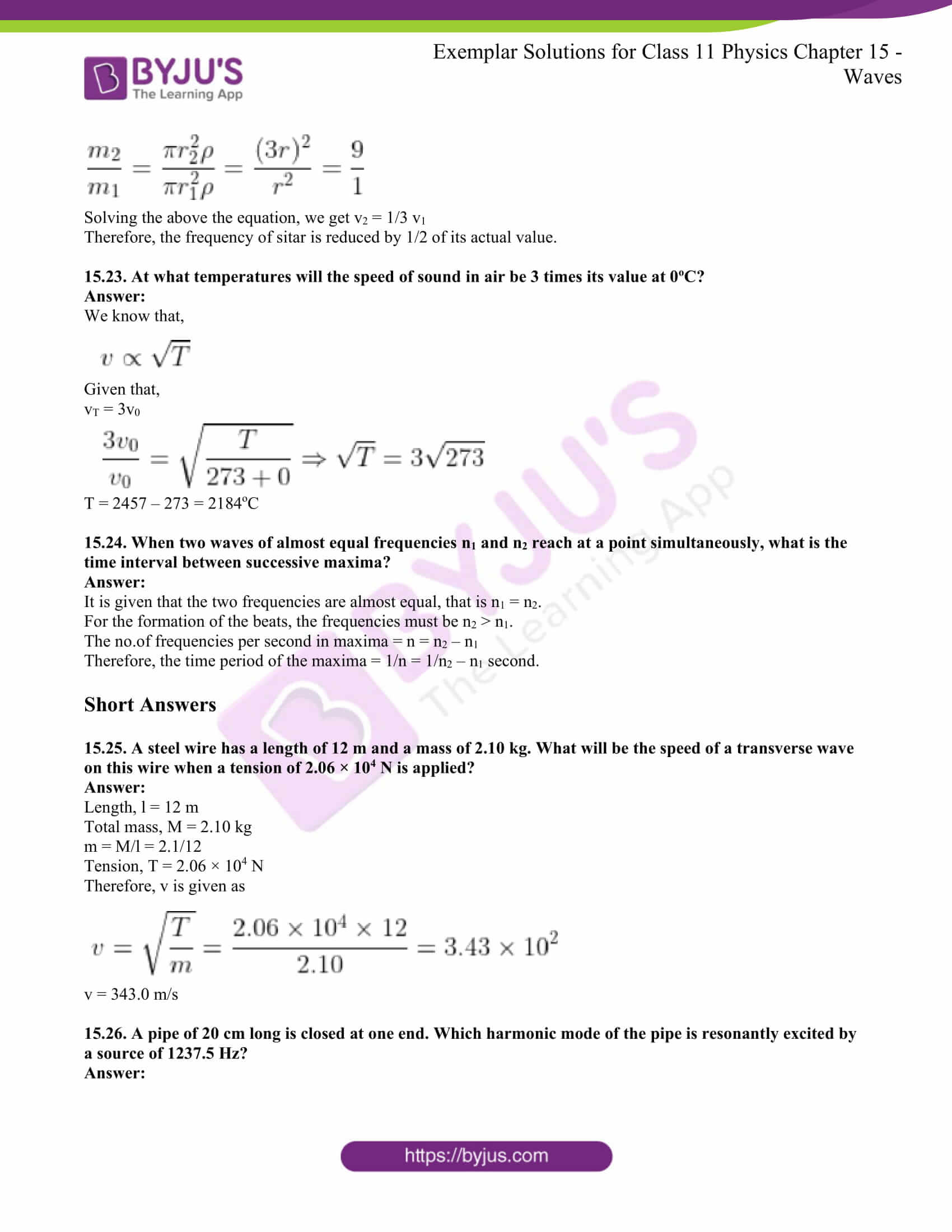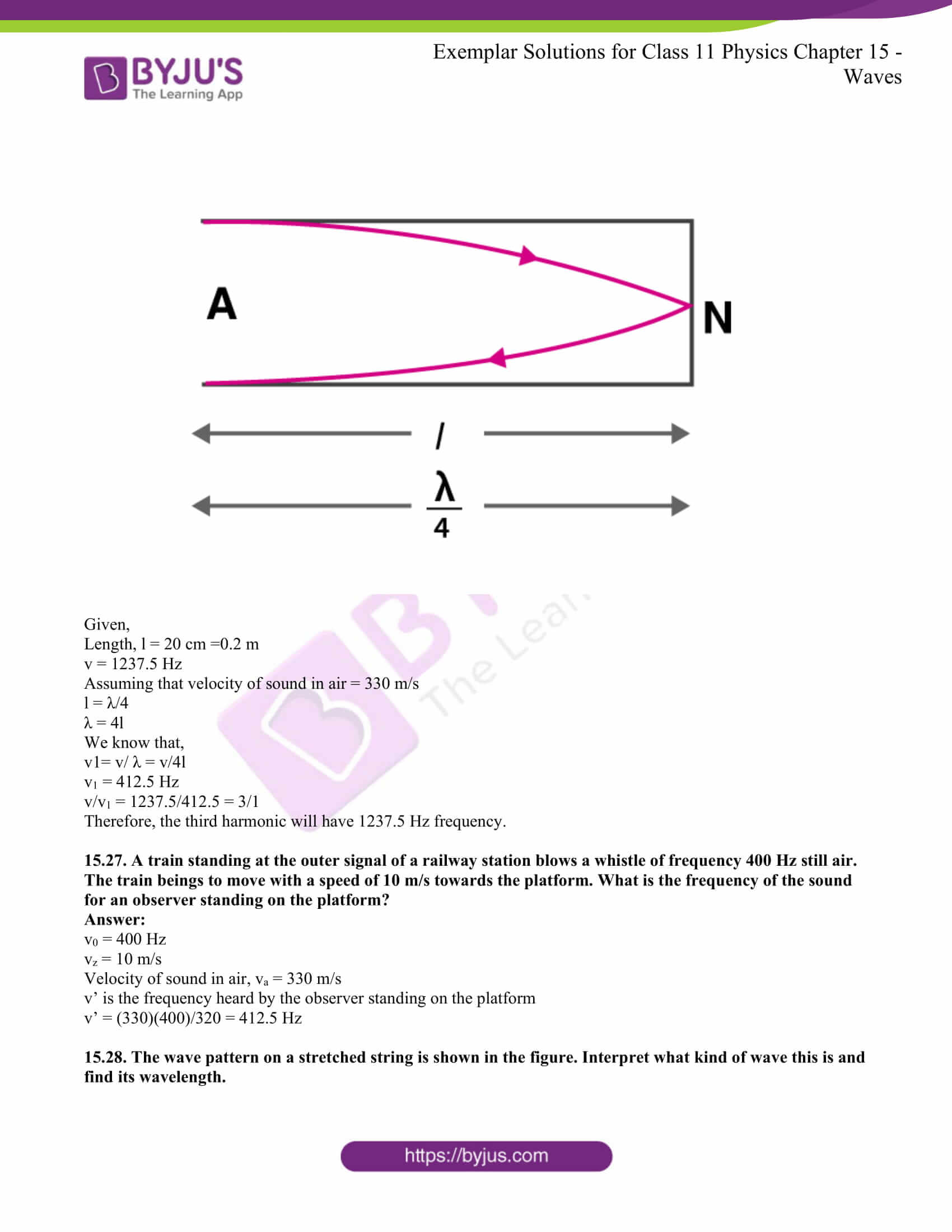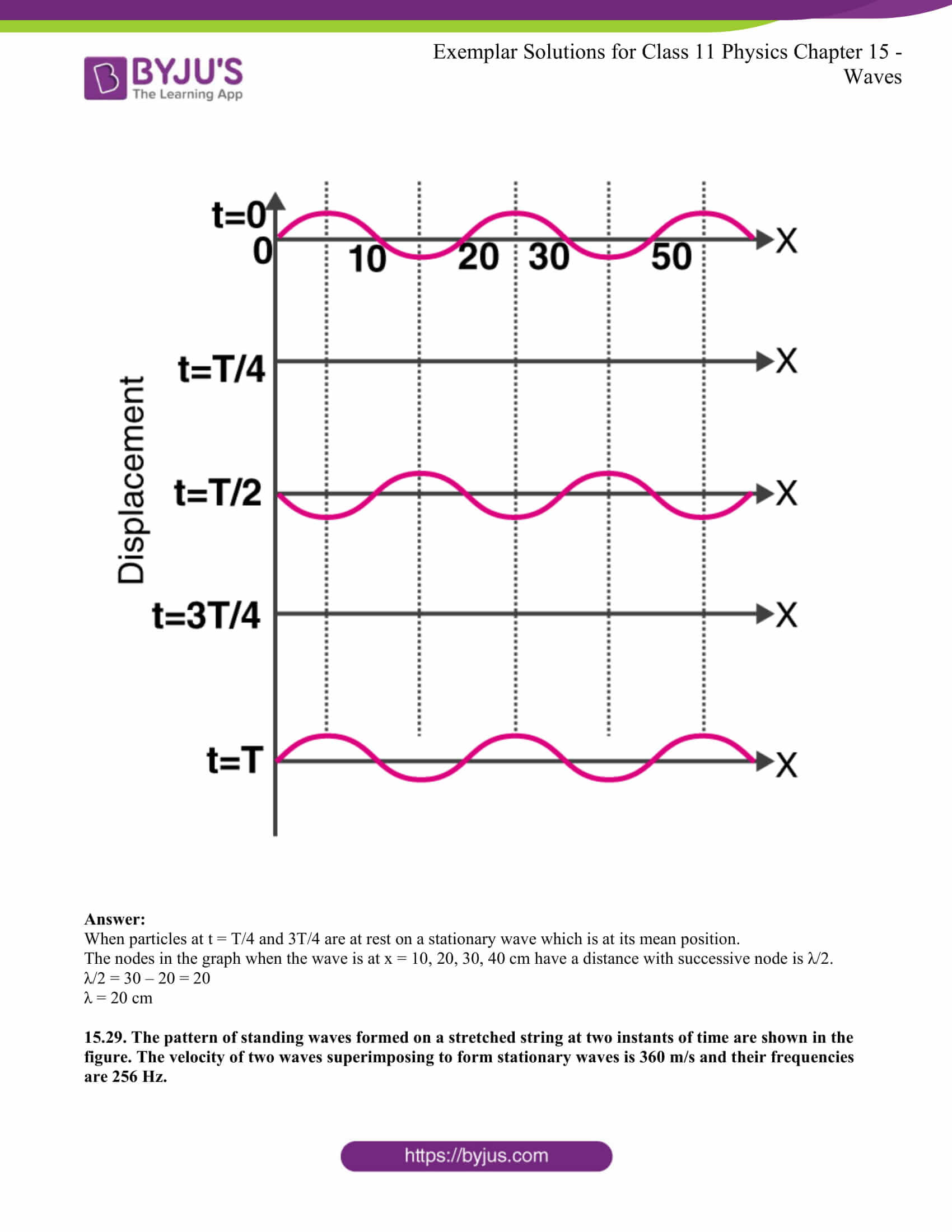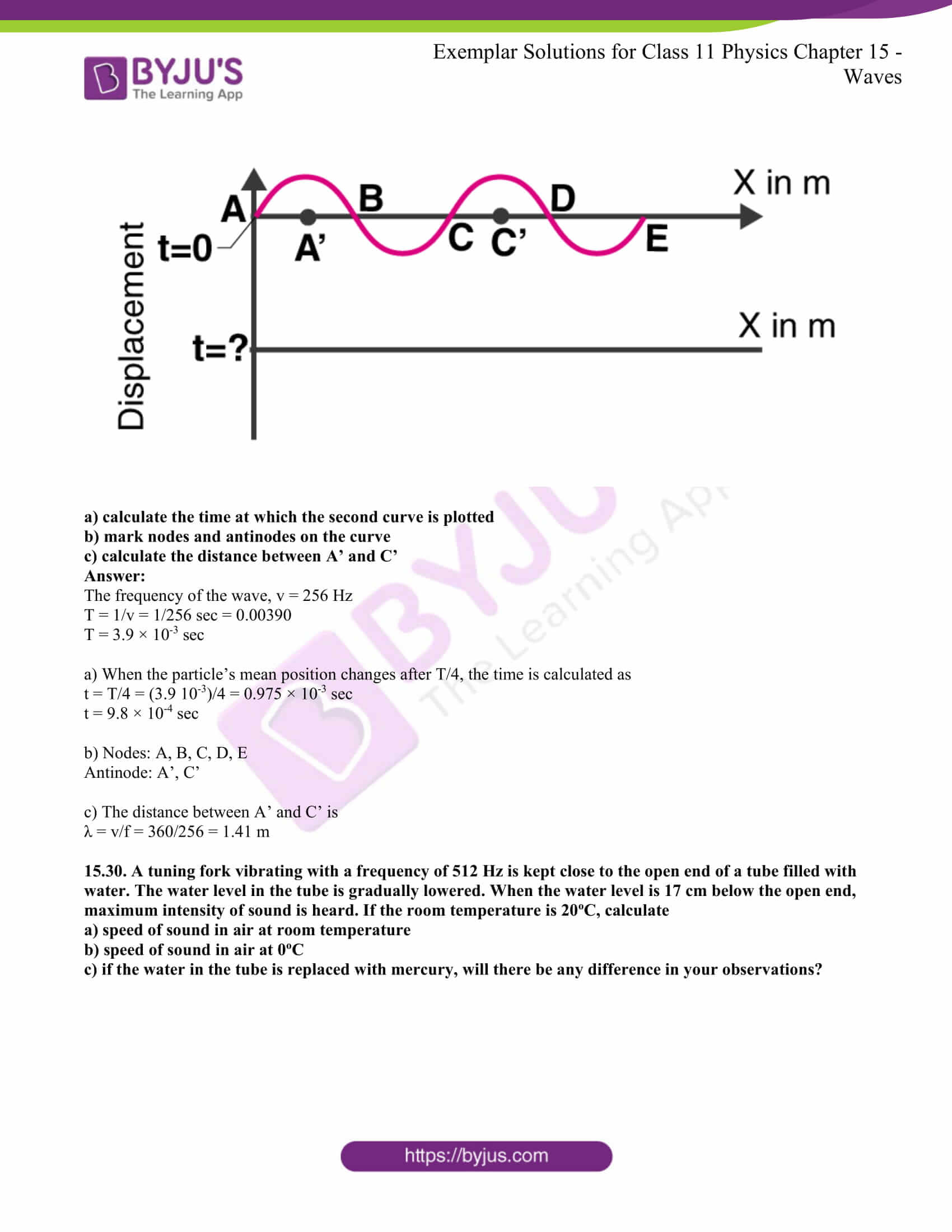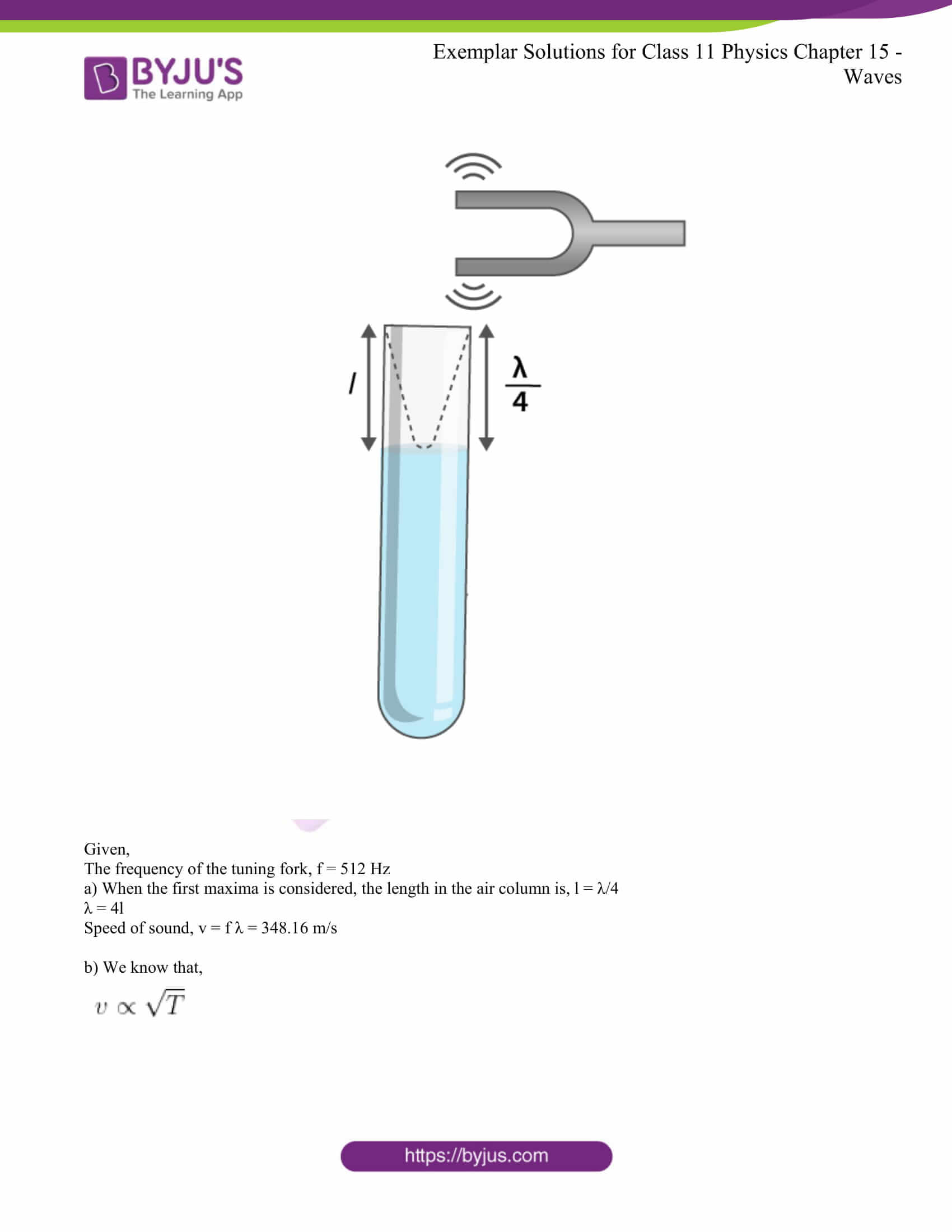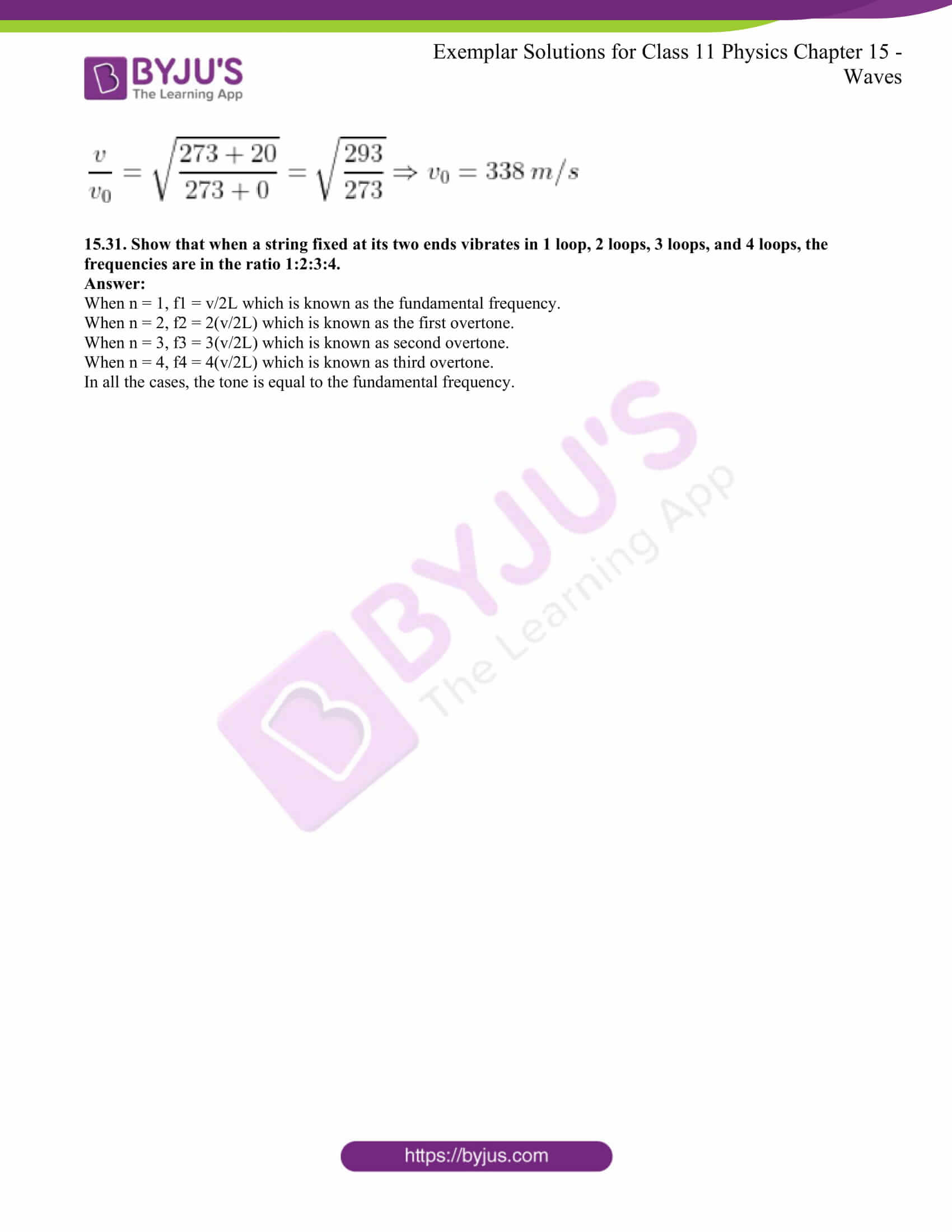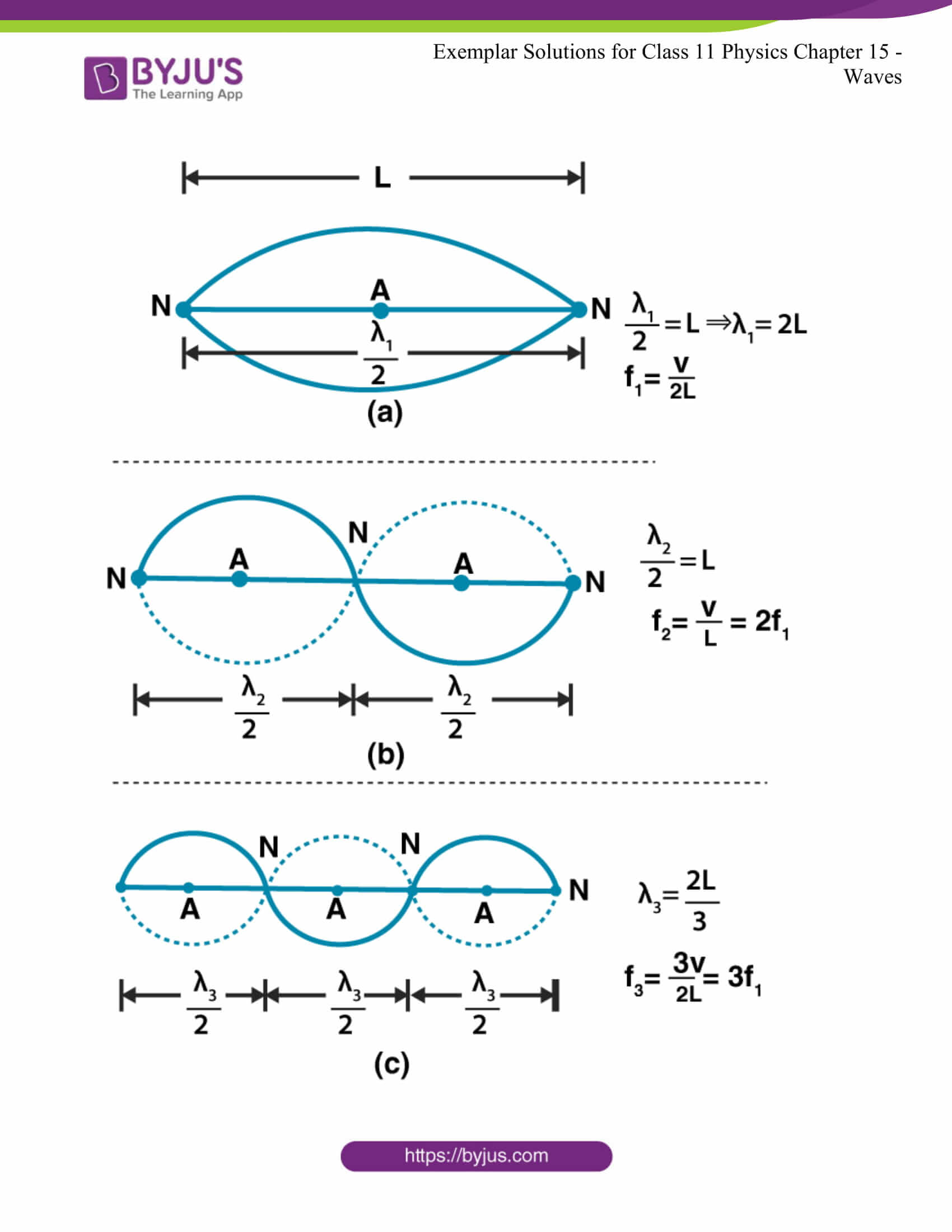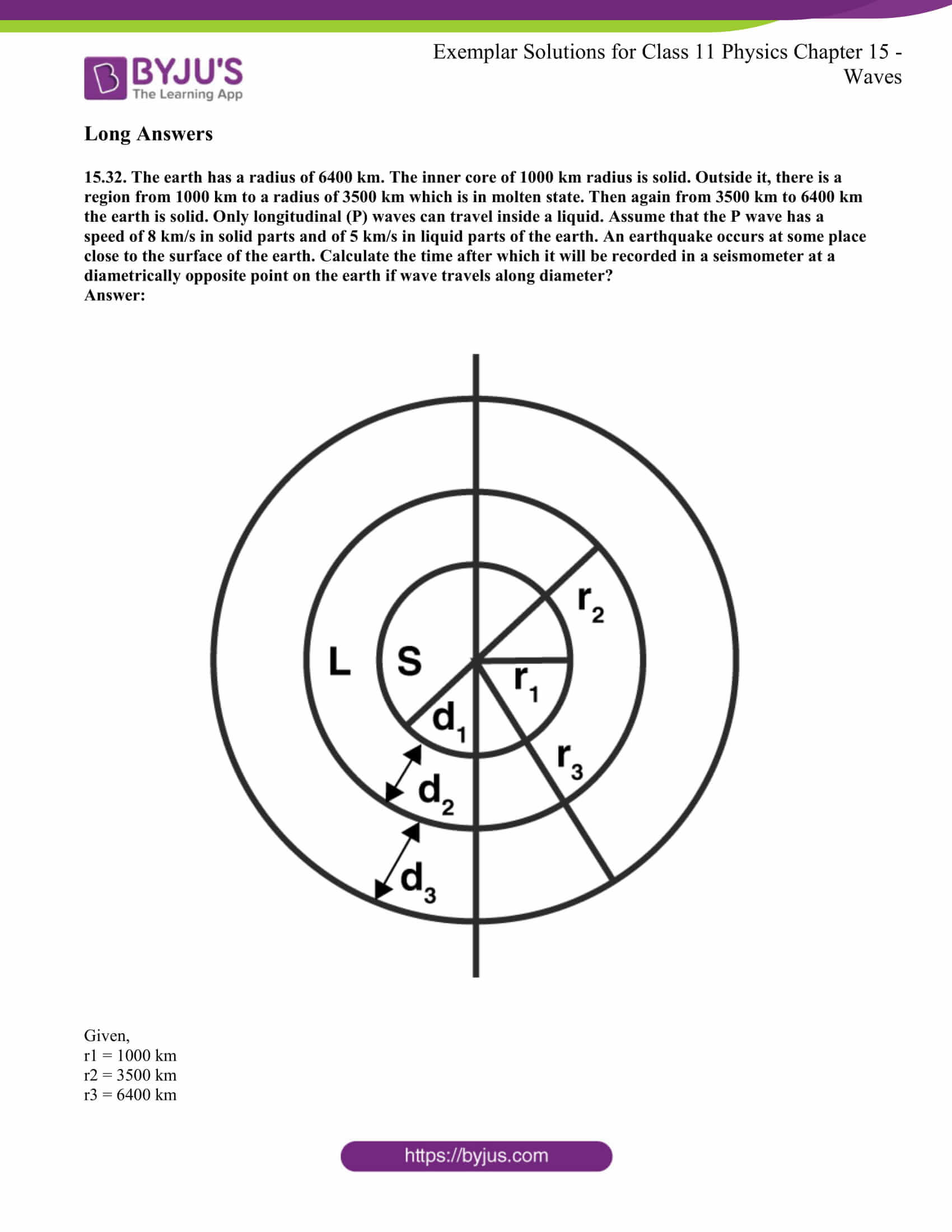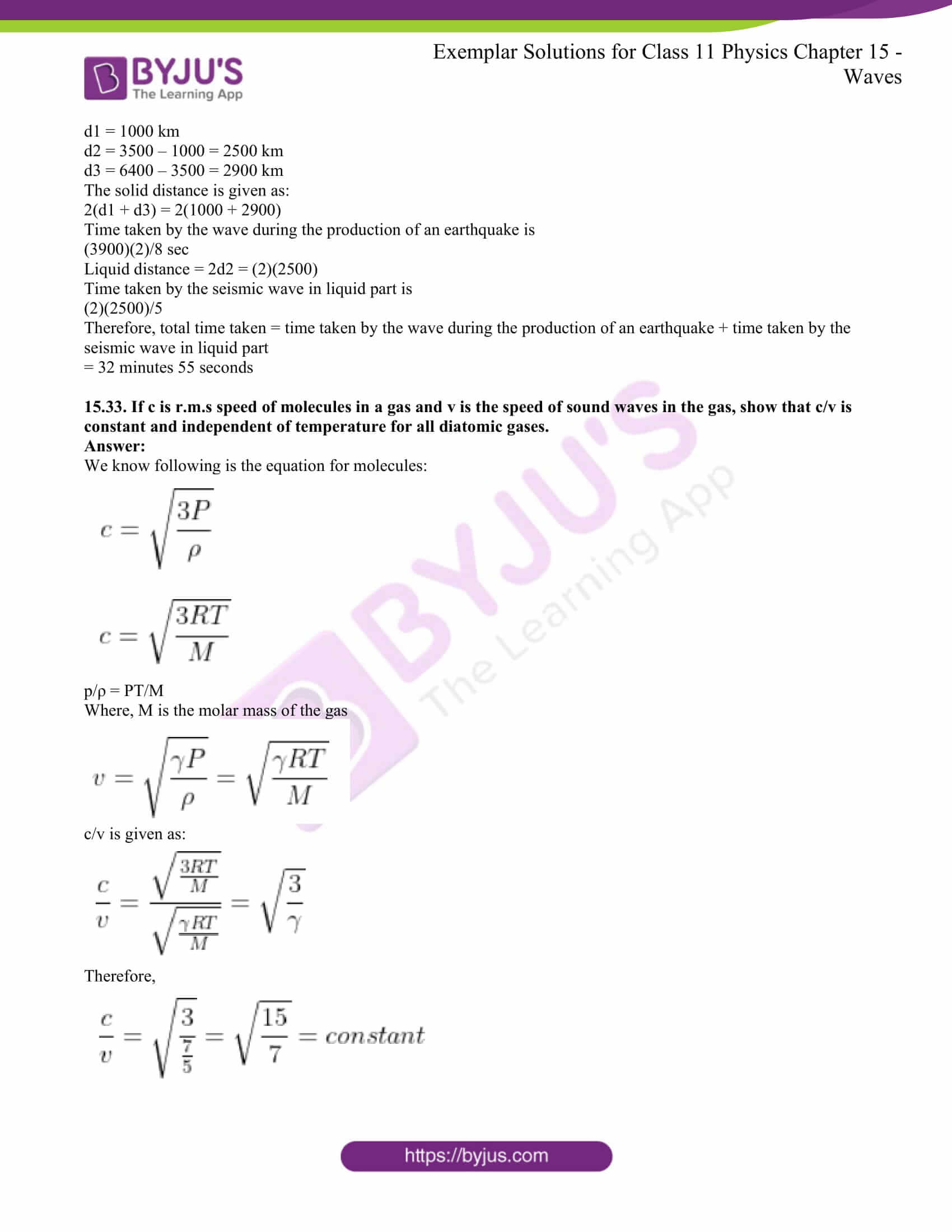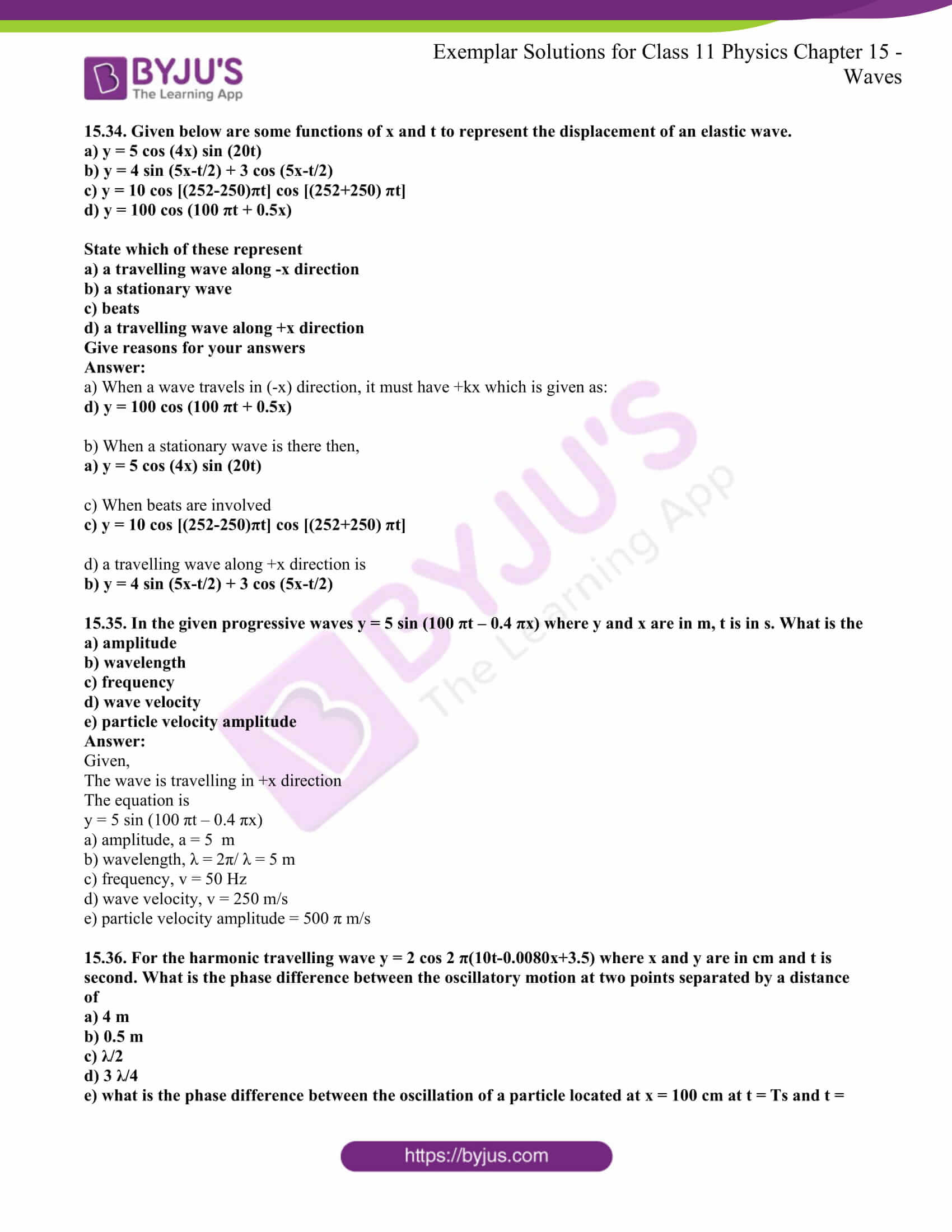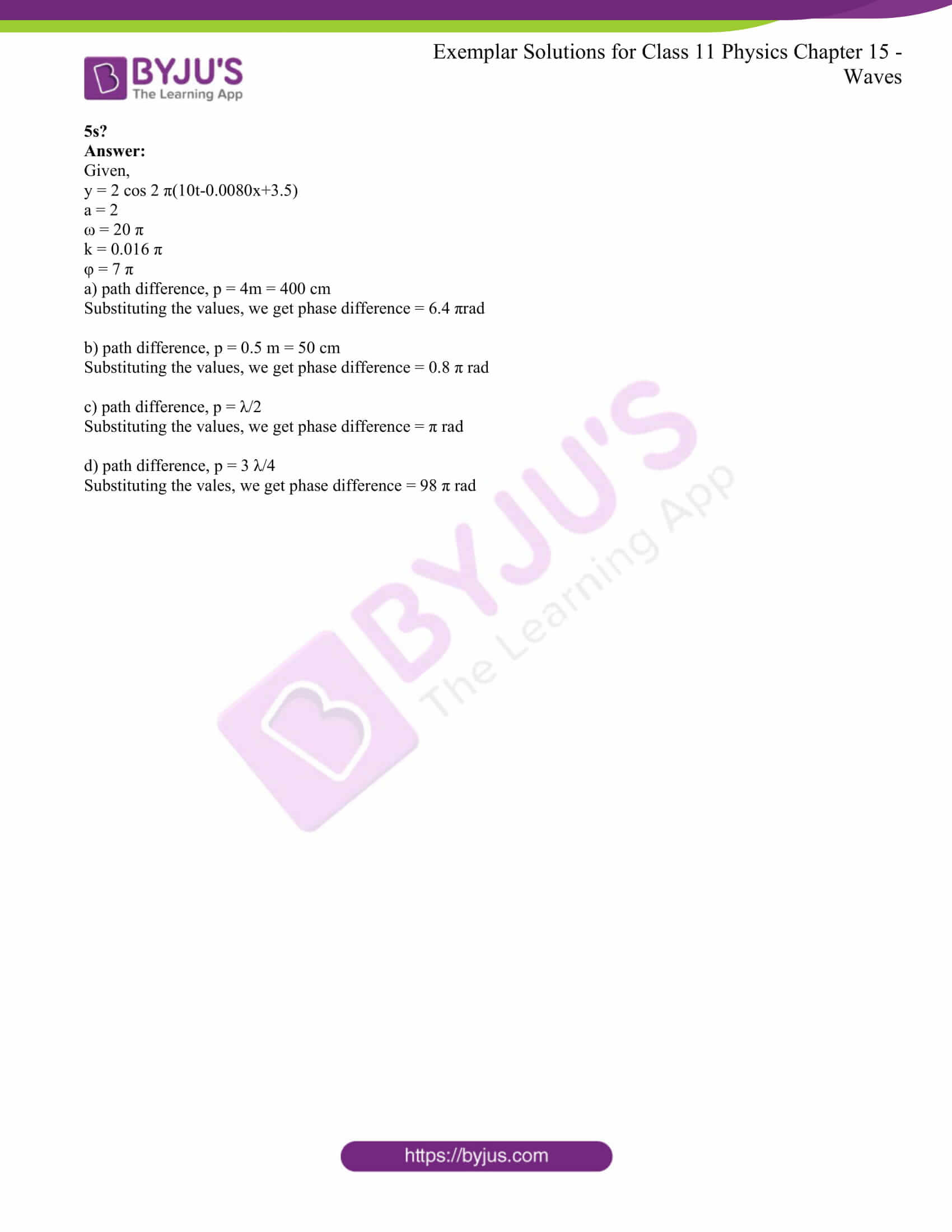### Multiple-choice Questions I

15.1. Water waves produced by a motorboat sailing in water are

a) neither longitudinal nor transverse

b) both longitudinal and transverse

c) only longitudinal

d) only transverse

The correct answer is b) both longitudinal and transverse

15.2. Sound waves of wavelength λ travelling in a medium with a speed of v m/s enter into another medium where its speed is 2v m/s. The wavelength of sound waves in the second medium is

a) λ

b) λ/2

c) 2 λ

d) 4 λ

The correct answer is c) 2 λ

15.3. Speed of sound wave in air

a) is independent of temperature

b) increases with pressure

c) increases with an increase in humidity

d) decreases with an increase in humidity

The correct answer is c) increases with an increase in humidity

15.4. Change in temperature of the medium changes

a) frequency of sound waves

b) amplitude of sound waves

c) wavelength of sound waves

d) loudness of sound waves

The correct answer is c) wavelength of sound waves

15.5. With the propagation of longitudinal waves through a medium, the quantity transmitted is

a) matter

b) energy

c) energy and matter

d) energy, matter, and momentum

The correct answer is b) energy

15.6. Which of the following statements are true for wave motion?

a) mechanical transverse waves can propagate through all mediums

b) longitudinal waves can propagate through solids only

c) mechanical transverse waves can propagate through solids only

d) longitudinal waves can propagate through a vacuum

The correct answer is c) mechanical transverse waves can propagate through solids only

15.7. A sound wave is passing through the air column in the form of compression and rarefaction. In consecutive compressions and rarefactions,

a) density remains constant

b) Boyle’s law is obeyed

c) bulk modulus of air oscillates

d) there is no transfer of heat

The correct answer is d) there is no transfer of heat

15.8. Equation of a plane progressive wave is given by y = 0.6 sin 2π(t-x/2). On reflection from a denser medium, its amplitude becomes 2/3 of the amplitude of the incident wave

a) y = 0.6 sin 2π(t+x/2)

b) y = -0.4 sin 2π(t+x/2)

c) y = 0.4 sin 2π(t+x/2)

d) y = -0.4 sin 2π(t-x/2)

The correct answer is b) y = -0.4 sin 2π(t+x/2)

15.9. A string of mass 2.5 kg is under a tension of 200 N. The length of the stretched string is 20.0 m. If the transverse jerk is struck at one end of the string, the disturbance will reach the other end in

a) one second

b) 0.5 second

c) 2 seconds

d) data given is insufficient

The correct answer is b) 0.5 second

15.10. A train whistling at constant frequency is moving towards a station at a constant speed V. The train goes past a stationary observer on the station. The frequency n’ of the sound as heard by the observer is plotted as a function of time t. Identify the expected curve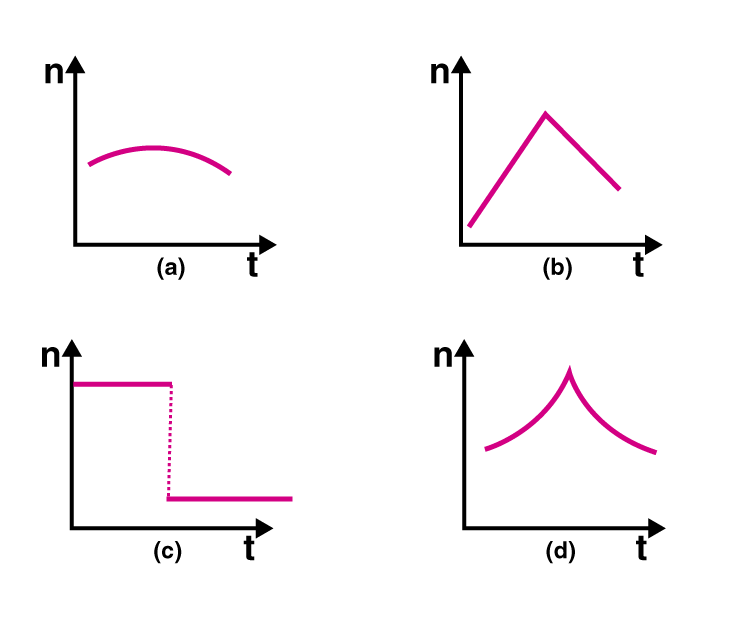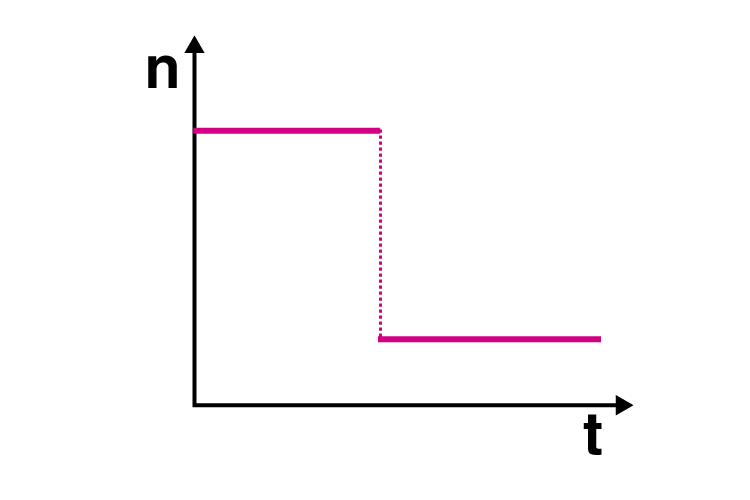### Multiple Choice Questions II

15.11. A transverse harmonic wave on a string is described by y (x,t) = 3.0 sin (36t + 0.018x + π/4) where x and y are in cm and t is in s. The positive direction of x is from left to right

a) the wave is travelling from right to left

b) the speed of the wave is 20 m/s

c) frequency of the wave is 5.7 Hz

d) the least distance between two successive crests in the wave is 2.5 cm

a) the wave is travelling from right to left

b) the speed of the wave is 20 m/s

c) frequency of the wave is 5.7 Hz

15.12. The displacement of a string is given by y(x,t) = 0.06 sin (2πx/3) cos (120πt) where x and y are in m and t in s. The length of the string is 1.5 m and its mass is 3.0 × 10-2kg.

a) it represents a progressive wave of frequency 60 Hz

b) it represents a stationary wave of frequency 60 Hz

c) it is the result of the superposition of two waves of wavelength 3 m, frequency 60 Hz each travelling with a speed of 180 m/s in the opposite direction

d) amplitude of this wave is constant

b) it represents a stationary wave of frequency 60 Hz

c) it is the result of the superposition of two waves of wavelength 3 m, frequency 60 Hz each travelling with a speed of 180 m/s in the opposite direction

15.13. Speed of sound waves in a fluid depends upon

a) directly on the density of the medium

b) square of bulk modulus of the medium

c) inversely on the square root of density

d) directly on the square root of bulk modulus of the medium

c) inversely on the square root of density

d) directly on the square root of bulk modulus of the medium

15.14. During propagation of a plane progressive mechanical wave

a) all the particles are vibrating in the same phase

b) amplitude of all the particles is equal

c) particles of the medium executes SHM

d) wave velocity depends upon the nature of the medium

b) amplitude of all the particles is equal

c) particles of the medium executes SHM

d) wave velocity depends upon the nature of the medium

15.15. The transverse displacement of a string is given by y(x,t) = 0.06 sin (2πx/3) cos (120πt). All the points on the string between two consecutive nodes vibrate with

a) same frequency

b) same phase

c) same energy

d) different amplitude

a) same frequency

b) same phase

d) different amplitude

15.16. A train, standing in a station yard, blows a whistle of frequency 400 Hz in still air. The wind starts blowing in the direction from the yard to the station with a speed of 10 m/s. Given that the speed of sound in still air is 340 m/s

a) the frequency of sound as heard by an observer standing on the platform is 400 Hz

b) the speed of sound for the observer standing on the platform is 350 m/s

c) the frequency of sound as heard by the observer standing on the platform will increase

d) the frequency of sound as heard by the observer standing on the platform will decrease

a) the frequency of sound as heard by an observer standing on the platform is 400 Hz

b) the speed of sound for the observer standing on the platform is 350 m/s

15.17. Which of the following statements is true for a stationary wave?

a) every particle has a fixed amplitude which is different from the amplitude of its nearest particle

b) all the particles cross their mean position at the same time

c) all the particles oscillate with the same amplitude

d) there is no net transfer of energy across any plane

e) there are some particles which are always at rest

a) every particle has a fixed amplitude which is different from the amplitude of its nearest particle

b) all the particles cross their mean position at the same time

d) there is no net transfer of energy across any plane

e) there are some particles which are always at rest

15.18. A sonometer wire is vibrating in resonance with a tuning fork. Keeping the tension applied the same, the length of the wire is doubled. Under what conditions would the tuning fork still be at resonance with the wire?

The length of the wire used in a sonometer is twice when it vibrates. Therefore, if the tuning fork resonates at L, the sonometer resonates at 2L. The following equation is used to express the frequency of the sonometer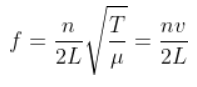The velocity of the wave from the sonometer is constant. The resonance between the tuning fork and the wire will remain even after the change in the length of the wire.

Then,

n/L = constant

Which also means that,

n1/L1 = n2/L2

n1/L1 = n2/2L2

n2 = 2n1

Therefore, when the wire length is doubled, the resonance also gets doubled.

15.19. An organ pipe of length L open at both ends is found to vibrate in its first harmonic when sounded with a tuning fork of 480 Hz. What should be the length of a pipe closed at one end, so that it also vibrates in its first harmonic with the same tuning fork?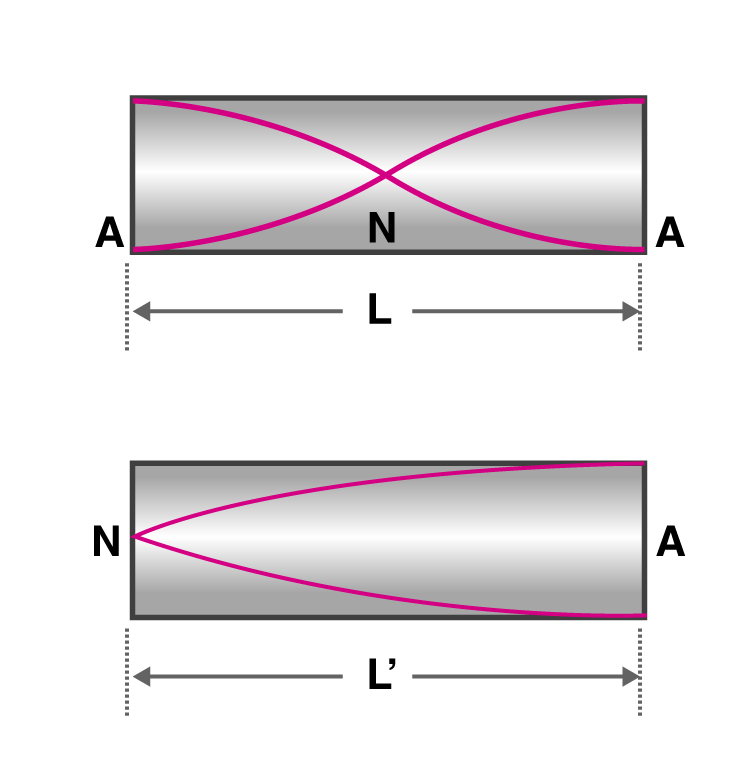From the harmonic is open, L = λ/2

λ = 2L

v/f0 = 2L

(f0)open = v/2L

where v is the speed of the sound wave in the air.

When the harmonic is closed, L’ = λ/4

λ = 4L’

(f0)closed = v/4L’

v/2L = v/4L’

L’ = L/2

15.20. A tuning fork A, marked 512 Hz, produces 5 beats per second, where sounded with another unmarked tuning fork B. If B is loaded with wax, the number of beats is again 5 per second. What is the frequency of the tuning fork B when not loaded?

When tuning fork B is loaded with the wax, the frequency of the tuning fork becomes less than its original frequency. When the tuning fork is marked 512 Hz, let’s assume that the tuning fork B is marked to 517 Hz because it produces 5 beats per second and when it is loaded, the frequency is less than 512 Hz. So it can be said that when the tuning fork is not loaded, the frequency remains the same, that is, 517 Hz.

15.21. The displacement of an elastic wave is given by the function y = 3 sin ωt + 4 cos ωt where y is in cm and t is in second. Calculate the resultant amplitude.

Given,

y = 3 sin ωt + 4 cos ωt

Let’s consider

3 = a cos φ

4 = a sin φ

Then

y = a cos φ ωt + a sin φ ωt

y = a sin (ωt + φ)

tan φ = 4/3

φ = tan-1 4/3

When the above equation is squared, we het

a2 = 25

a = 5

Therefore, the new amplitude is 5 cm.

15.22. A sitar wire is replaced by another wire of the same length and material but of three times the earlier radius. If the tension in the wire remains the same, by what factor will the frequency change?

The frequency of the stretched wire is given as: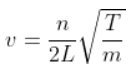Given that,

No.of harmonic n, length L, and tension T are the same in both cases.

Therefore,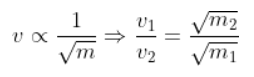Substituting the values of mass per unit length, we get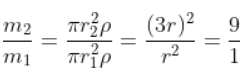Solving the above the equation, we get v2 = 1/3 v1

Therefore, the frequency of the sitar is reduced by 1/2 of its actual value.

15.23. At what temperatures will the speed of sound in the air be 3 times its value at 0oC?

We know that,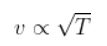Given that,

vT = 3v0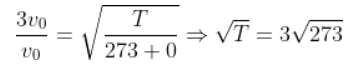T = 2457 – 273 = 2184oC

15.24. When two waves of almost equal frequencies, n1 and n2, reach at a point simultaneously, what is the time interval between successive maxima?

It is given that the two frequencies are almost equal, that is n1 = n2.

For the formation of the beats, the frequencies must be n2 > n1.

The no.of frequencies per second in maxima = n = n2 – n1

Therefore, the time period of the maxima = 1/n = 1/n2 – n1 second.

15.25. A steel wire has a length of 12 m and a mass of 2.10 kg. What will be the speed of a transverse wave on this wire when a tension of 2.06 × 104 N is applied?

Length, l = 12 m

Total mass, M = 2.10 kg

m = M/l = 2.1/12

Tension, T = 2.06 × 104 N

Therefore, v is given as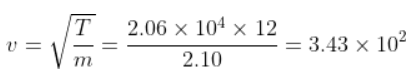v = 343.0 m/s

15.26. A pipe of 20 cm long is closed at one end. Which harmonic mode of the pipe is resonantly excited by a source of 1237.5 Hz?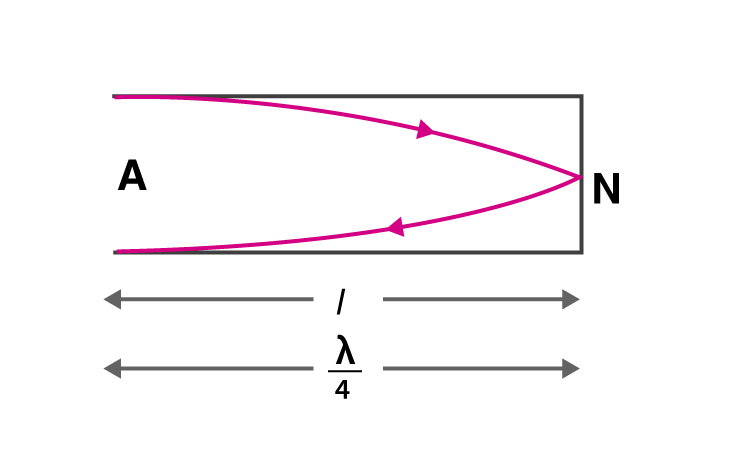Given,

Length, l = 20 cm =0.2 m

v = 1237.5 Hz

Assuming that velocity of sound in air = 330 m/s

l = λ/4

λ = 4l

We know that,

v1= v/ λ = v/4l

v1 = 412.5 Hz

v/v1 = 1237.5/412.5 = 3/1

Therefore, the third harmonic will have 1237.5 Hz frequency.

15.27. A train standing at the outer signal of a railway station blows a whistle of frequency 400 Hz still air. The train beings to move at a speed of 10 m/s towards the platform. What is the frequency of the sound for an observer standing on the platform?

v0 = 400 Hz

vz = 10 m/s

The velocity of sound in air, va = 330 m/s

v’ is the frequency heard by the observer standing on the platform

v’ = (330)(400)/320 = 412.5 Hz

15.28. The wave pattern on a stretched string is shown in the figure. Interpret what kind of wave this is and find its wavelength.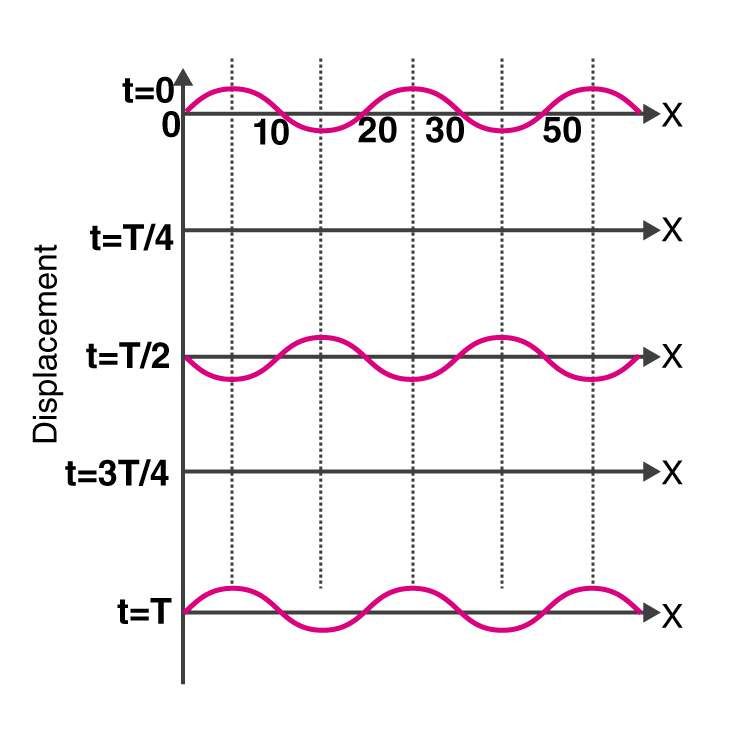When particles at t = T/4 and 3T/4 are at rest on a stationary wave which is at its mean position.

The nodes in the graph when the wave is at x = 10, 20, 30, 40 cm have a distance with the successive node is λ/2.

λ/2 = 30 – 20 = 20

λ = 20 cm

15.29. The pattern of standing waves formed on a stretched string at two instants of time is shown in the figure. The velocity of two waves superimposing to form stationary waves is 360 m/s, and their frequencies are 256 Hz.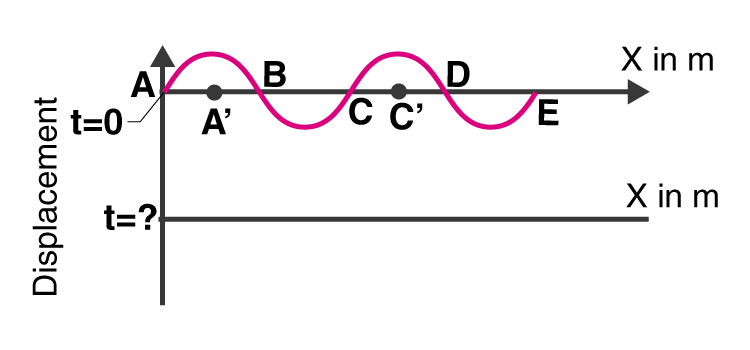a) calculate the time at which the second curve is plotted

b) mark nodes and antinodes on the curve

c) calculate the distance between A’ and C’

The frequency of the wave, v = 256 Hz

T = 1/v = 1/256 sec = 0.00390

T = 3.9 × 10-3 sec

a) When the particle’s mean position changes after T/4, the time is calculated as

t = T/4 = (3.9 10-3)/4 = 0.975 × 10-3 sec

t = 9.8 × 10-4 sec

b) Nodes: A, B, C, D, E

Antinode: A’, C’

c) The distance between A’ and C’ is

λ = v/f = 360/256 = 1.41 m

15.30. A tuning fork vibrating with a frequency of 512 Hz is kept close to the open end of a tube filled with water. The water level in the tube is gradually lowered. When the water level is 17 cm below the open end, the maximum intensity of sound is heard. If the room temperature is 20oC, calculate

a) speed of sound in air at room temperature

b) speed of sound in air at 0oC

c) if the water in the tube is replaced with mercury, will there be any difference in your observations?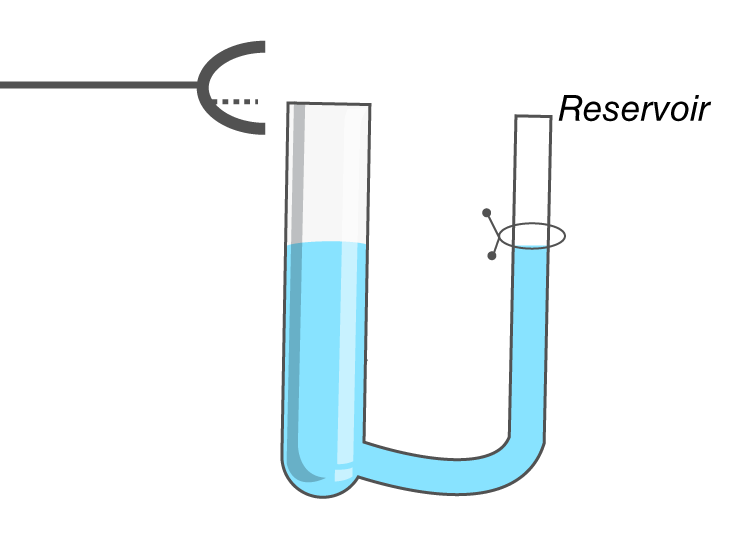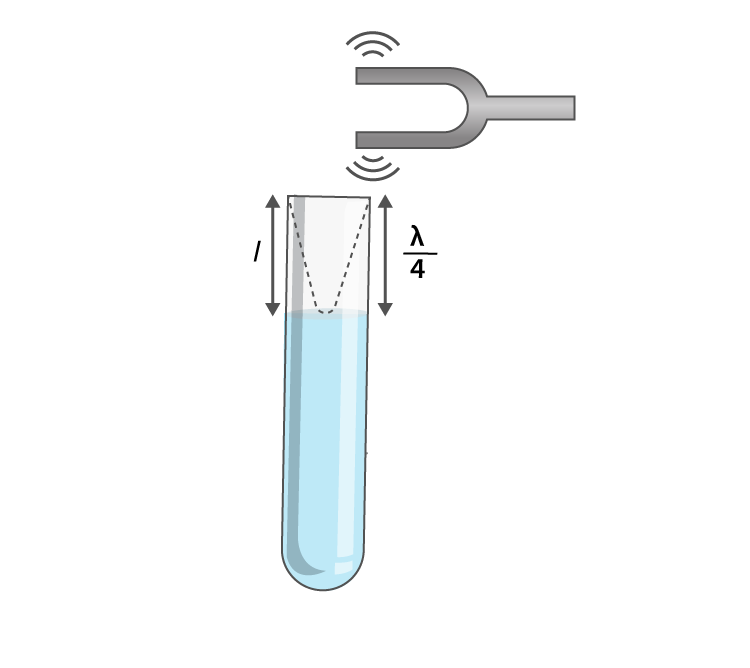Given,

The frequency of the tuning fork, f = 512 Hz

a) When the first maxima is considered, the length in the air column is, l = λ/4

λ = 4l

Speed of sound, v = f λ = 348.16 m/s

b) We know that,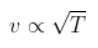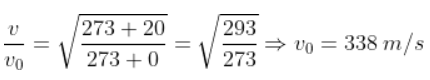15.31. Show that when a string fixed at its two ends vibrates in 1 loop, 2 loops, 3 loops, and 4 loops, the frequencies are in the ratio 1:2:3:4.

When n = 1, f1 = v/2L, which is known as the fundamental frequency.

When n = 2, f2 = 2(v/2L), which is known as the first overtone.

When n = 3, f3 = 3(v/2L), which is known as the second overtone.

When n = 4, f4 = 4(v/2L), which is known as the third overtone.

In all cases, the tone is equal to the fundamental frequency.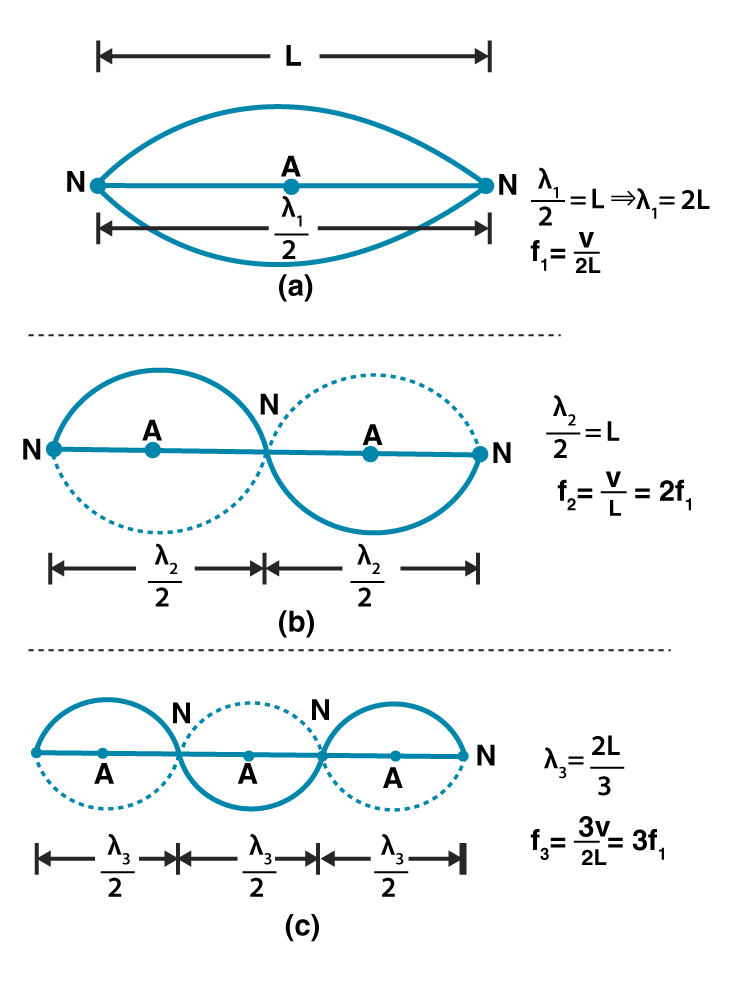15.32. The earth has a radius of 6400 km. The inner core of the 1000 km radius is solid. Outside it, there is a region from 1000 km to a radius of 3500 km which is in a molten state. Then again, from 3500 km to 6400 km, the earth is solid. Only longitudinal (P) waves can travel inside a liquid. Assume that the P wave has a speed of 8 km/s in solid parts and of 5 km/s in liquid parts of the earth. An earthquake occurs at someplace close to the surface of the earth. Calculate the time after which it will be recorded in a seismometer at a diametrically opposite point on the earth if the wave travels along diameter.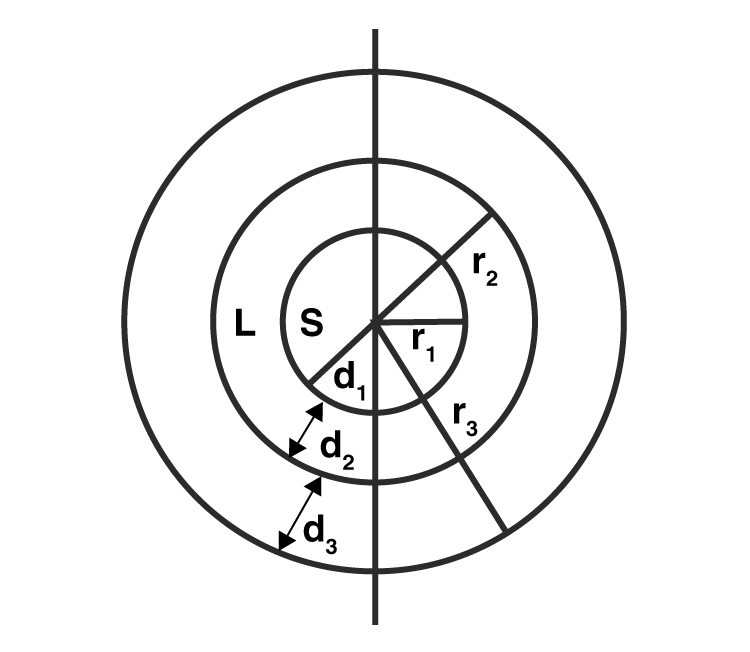Given,

r1 = 1000 km

r2 = 3500 km

r3 = 6400 km

d1 = 1000 km

d2 = 3500 – 1000 = 2500 km

d3 = 6400 – 3500 = 2900 km

The solid distance is given as:

2(d1 + d3) = 2(1000 + 2900)

Time taken by the wave during the production of an earthquake is

(3900)(2)/8 sec

Liquid distance = 2d2 = (2)(2500)

Time taken by the seismic wave in the liquid part is

(2)(2500)/5

Therefore, total time taken = time taken by the wave during the production of an earthquake + time taken by the seismic wave in the liquid part

= 32 minutes 55 seconds

15.33. If c is r.m.s speed of molecules in a gas and v is the speed of sound waves in the gas, show that c/v is constant and independent of temperature for all diatomic gases.

We know the following is the equation for molecules: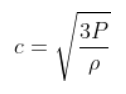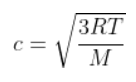p/ρ = PT/M

Where, M is the molar mass of the gas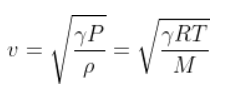c/v is given as: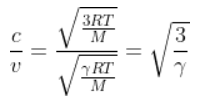Therefore,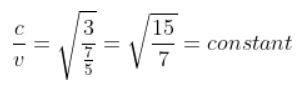15.34. Given below are some functions of x and t to represent the displacement of an elastic wave.

a) y = 5 cos (4x) sin (20t)

b) y = 4 sin (5x-t/2) + 3 cos (5x-t/2)

c) y = 10 cos [(252-250)πt] cos [(252+250) πt]

d) y = 100 cos (100 πt + 0.5x)

State which of these represent

a) a travelling wave along -x direction

b) a stationary wave

c) beats

d) a travelling wave along +x direction

a) When a wave travels in (-x) direction, it must have +kx, which is given as:

d) y = 100 cos (100 πt + 0.5x)

b) When a stationary wave is there, then,

a) y = 5 cos (4x) sin (20t)

c) When beats are involved

c) y = 10 cos [(252-250)πt] cos [(252+250) πt]

d) a travelling wave along +x direction is

b) y = 4 sin (5x-t/2) + 3 cos (5x-t/2)

15.35. In the given progressive waves y = 5 sin (100 πt – 0.4 πx) where y and x are in m, t is in s. What is the

a) amplitude

b) wavelength

c) frequency

d) wave velocity

e) particle velocity amplitude

Given,

The wave is travelling in +x direction

The equation is

y = 5 sin (100 πt – 0.4 πx)

a) amplitude, a = 5 m

b) wavelength, λ = 2π/ λ = 5 m

c) frequency, v = 50 Hz

d) wave velocity, v = 250 m/s

e) particle velocity amplitude = 500 π m/s

15.36. For the harmonic travelling wave y = 2 cos 2 π(10t-0.0080x+3.5) where x and y are in cm and t is second. What is the phase difference between the oscillatory motion at two points separated by a distance of

a) 4 m

b) 0.5 m

c) λ/2

d) 3 λ/4

e) what is the phase difference between the oscillation of a particle located at x = 100 cm at t = Ts and t = 5s?

Given,

y = 2 cos 2 π(10t-0.0080x+3.5)

a = 2

ω = 20 π

k = 0.016 π

φ = 7 π

a) path difference, p = 4m = 400 cm

Substituting the values, we get phase difference = 6.4 πrad

b) path difference, p = 0.5 m = 50 cm

Substituting the values, we get phase difference = 0.8 π rad

c) path difference, p = λ/2

Substituting the values, we get phase difference = π rad

d) path difference, p = 3 λ/4

Substituting the values, we get phase difference = 98 π rad

1. Microwaves
3. Gamma rays
4. X-rays

#### Important Concepts Covered in Class 11 Physics Chapter 15 Waves

1. Transverse and longitudinal waves
2. Displacement relation in a progressive wave
3. The speed of a travelling wave
4. The principle of superposition of waves
5. Reflection of waves
6. Beats
7. Doppler effect.

### Why Opt for BYJU’S?

Waves is an important topic which also lays the foundation for some of the topics of CBSE Class 12, so students are advised to study this topic thoroughly in order to avoid difficulty in future. To help you understand this topic, BYJU’S brings videos, animations, previous years’ question papers, sample papers and other study materials that will not only help you understand the topic clearly but also memorise the topic for a longer period of time.